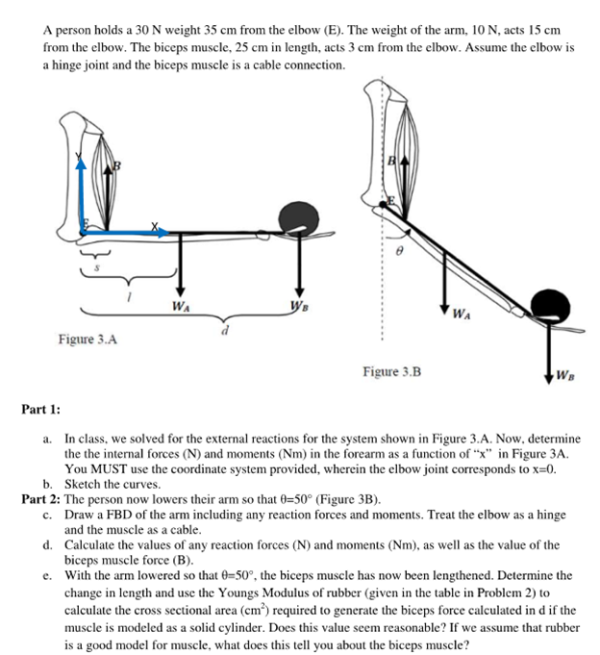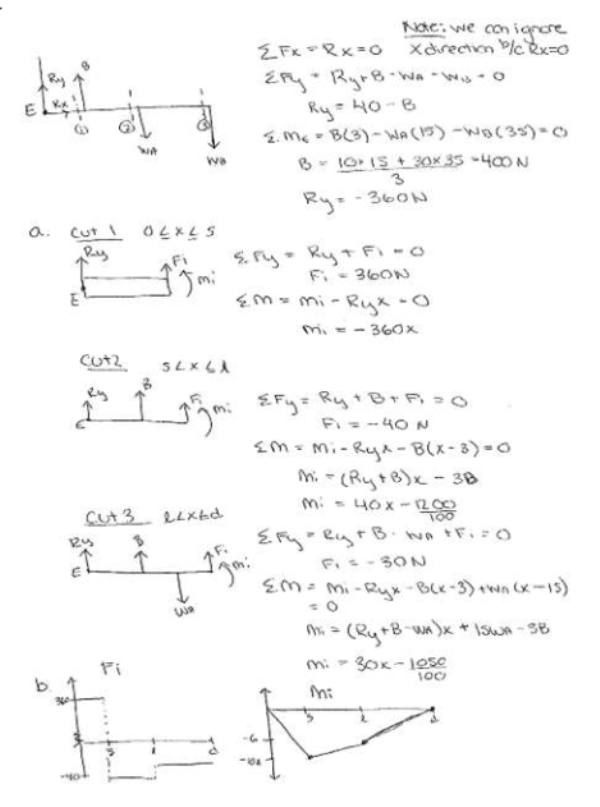A person holds a 30 N weight 35 cm from the elbow E. The weight of the arm, 10 N, acts 15 cm from the elbow. The biceps muscle, 25 cm in length, acts 3 cm from the elbow. Assume the elbow is a hinge joint and the biceps muscle is a cable connection. a) Determine the internal forces and moments in the forearm as a function of x. b) sketch the curves. c) Draw a FBD of the arm including any reaction forces and moments. Treat the elbow as a hinge and the muscle as a cable. d) Calculate the values of any reaction forces and moments, as well as the value of the biceps muscle force. e) With the arm lowered so that theta=50 degrees, the biceps muscle has now been lengthened. Determine the change in length and use the Young's Modulus of rubber to calculate the cross sectional area required to generate the biceps force calculated in d if the muscle is modeled as a solid cylinder.A person holds a 30 N weight 35 cm from the elbow E. The weight of the arm, 10 N, acts 15 cm from the elbow. The biceps muscle, 25 cm in length, acts 3 cm from the elbow. Assume the elbow is a hinge joint and the biceps muscle is a cable connection. a) Determine the internal forces and moments in the forearm as a function of x. b) sketch the curves. c) Draw a FBD of the arm including any reaction forces and moments. Treat the elbow as a hinge and the muscle as a cable. d) Calculate the values of any reaction forces and moments, as well as the value of the biceps muscle force. e) With the arm lowered so that theta=50 degrees, the biceps muscle has now been lengthened. Determine the change in length and use the Young's Modulus of rubber to calculate the cross sectional area required to generate the biceps force calculated in d if the muscle is modeled as a solid cylinder.

biomechanics page 1 biomechanics biomechanics biomechanics biomechanics biomechanics biomechanics biomechanics biomechanics page 2